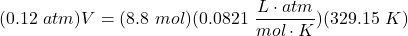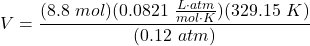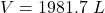How much volume will 8.8 moles of gas fill at 0.12 atm at 56*C

Question

How much volume will 8.8 moles of gas fill at 0.12 atm at 56*C

in progress 0
5 months 2021-09-02T07:29:32+00:00 1 Answers 0 views 0

2000 L

General Formulas and Concepts:

Atomic Structure

• Moles
• Temperature Conversion: K = °C + 273.15

Gas Laws

Ideal Gas Law: PV = nRT

• P is pressure
• V is volume (in L)
• n is number of moles
• R is gas constant
• T is temperature (in K)

Explanation:

Step 1: Define

[Given] 8.8 moles gas

[Given] 0.12 atm

[Given] 56 °C = 329.15 K

Step 2: Solve for V

1. Substitute in variables [Ideal Gas Law Formula]:2. Isolate V:3. Multiply/Divide [Cancel out units]:Step 3: Check

Follow sig fig rules and round. We are given 2 sig figs.

1981.7 L ≈ 2000 L